Home > ACC7 > Chapter cc28 > Lesson cc28.2.2 > Problem8-49

8-49.
1. Solve these equations for x. Check your answers. Homework Help ✎

1. 2(x + 4.5) = 32

2. 6 + 2.5x = 21

3.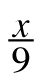=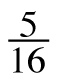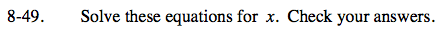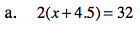Distribute.

2(x) + 2(4.5) = 32

2x + 9 = 32
−9 −9
2x = 23

Subtract 9 from both sides.

Divide by 2.

$\frac{2\textit{x}}{2}=\frac{23}{2}$

x = 11.5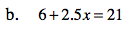x = 6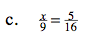Multiply both sides by 9 and by 16.